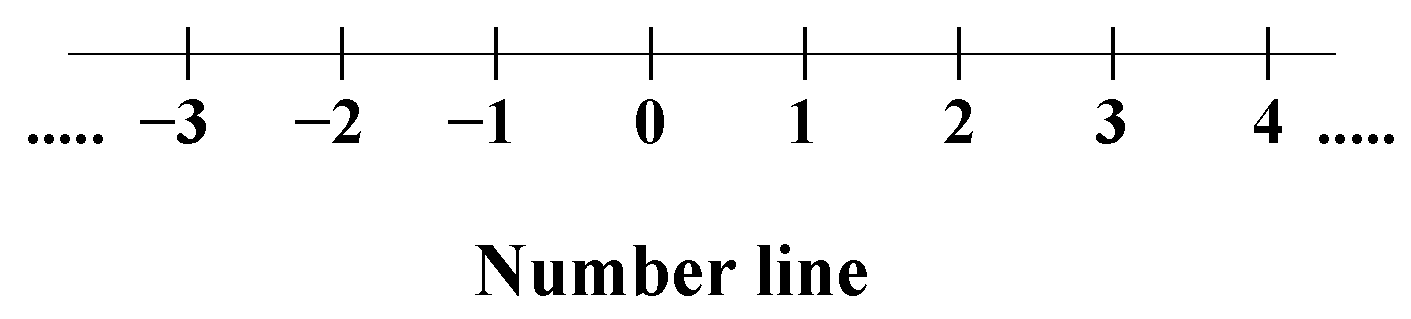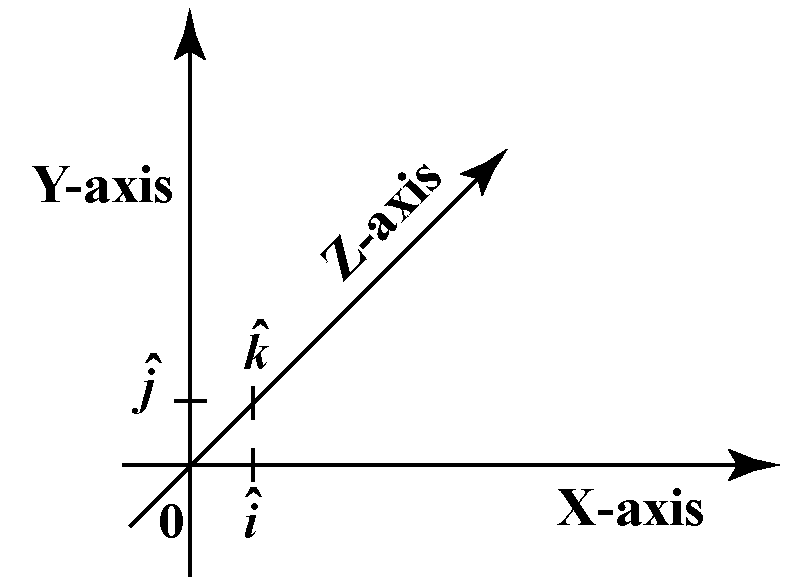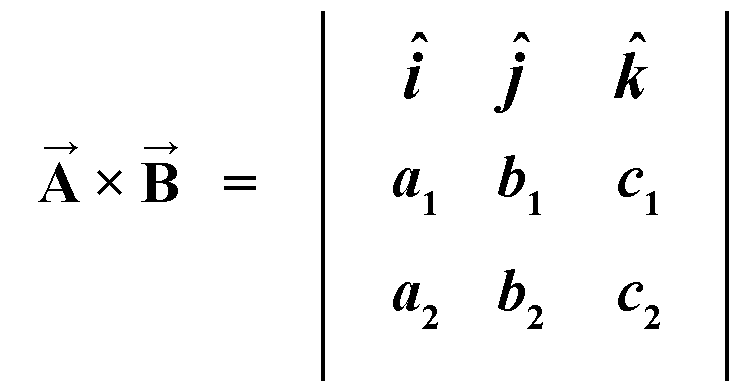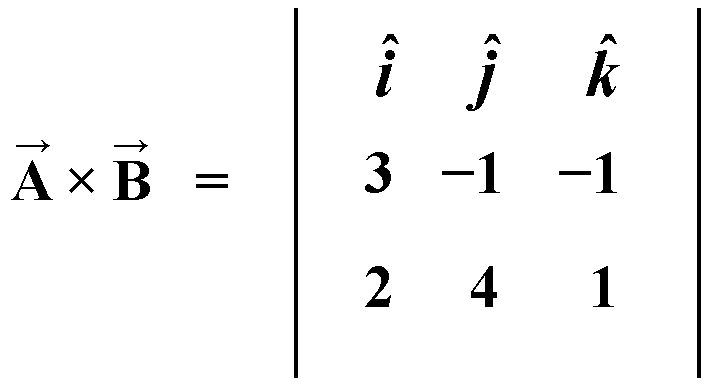# Vectors

The use of vectors started in the late 19th century with the advent of the field of electromagnetic induction.  Electromagnetic induction involves an interplay of electric forces and magnetic forces.  Here vectors are helpful to represent and perform calculations involving these forces.  In our daily life, you may think of vectors being used to represent the velocity of an aircraft, where both the speed and the direction of movement of the aircraft are to be known.In this mini-lesson, we shall explore the world of vectors, by finding answers to questions like what are vectors,  what are scalars, what is the difference between scalar and vectors, the magnitude of a vector, operations on vectors,  and properties of a vector.

## Lesson Plan

 1 What Are Vectors? 2 Challenging Question on Vectors 3 Think-Tank 4 Solved Examples on Vectors 5 Interactive Questions on Vectors

## What Are Vectors?

Vectors are quantities with both magnitude and direction.  Vectors help to simultaneously represent different quantities in the same expression.

The standard form of representation of a vector is:

$\vec A = a\hat i + b \hat j +c \hat k$

a, b, c are numeric values and $$\hat i, \hat j, \hat k$$ are the unit vectors along the x-axis, y-axis, and z-axis respectively.

All the normal operations of addition, subtraction, multiplication can be performed on vectors.

Vectors are used to represent quantities in physics, such as velocity, acceleration, force, or torque.

## What Are Scalars?

Scalars are simple numeric values representing the magnitude.  All the algebraic operations can be performed on scalars.

A few examples of scalars is the age of a person, the value x = 5, the marks of a student.

## What Is the Difference Between Scalars and Vectors?

Scalar has only magnitude, and vectors have both magnitude and direction.

A scalar can be represented on a number line.  The scalar can have both positive and negative values.A vector can be geometrically represented in a three-dimensional plane.A scalar can represent only one quantity at a time.  A vector can represent numerous interrelated quantities at the same time.

## The Magnitude Of a Vector

The magnitude of $$\hat A$$ is the numeric value of the vector and is represented as $$|A|$$

$|\vec A| = \sqrt{ (a^2 + b^2 + c^2) }$

Geometrically it is the distance of the vector $$\vec A$$ from the origin.

The magnitude of a vector is a scalar value.

## Operations on Vectors

Addition of Vectors: The vector addition is similar to scalar addition.  The individual components of the respective vectors are added to get the final value

$(a_1 \hat i + b_1\hat j + c_1\hat k) + (a_2\hat i + b_2\hat j + c_2\hat k) = (a_1 + a_2)\hat i +(b_1 + b_2)\hat j + (c_1 + c_2)\hat k$

Subtraction of Vectors: Subtraction is similar to the addition of vectors.  But here only the sign of one of the vectors is changed in direction and added to the other vector.

$(a_1\hat i + b_1\hat j + c_1\hat k) - (a_2\hat i + b_2\hat j + c_2\hat k) = (a_1 - a_2)\hat i +(b_1 - b_2)\hat j + (c_1 - c_2)\hat k$

Dot Product: The individual components of a vector are multiplied and the result is added to get the dot product of two vectors.

$(a_1 \hat i + b_1 \hat j + c_1 \hat k) . (a_2 \hat i + b_2 \hat j + c_2 \hat k) = (a_1 .a_2)+(b_1. b_2) + (c_1. c_2)$

Also  $$\vec A \times \vec B = |\vec A|.| \vec B|. Cos \theta$$

The resultant of a dot product of two vectors is a scalar value.

Cross Product: The vector components are represented in a matrix and a determinant of the matrix represents the result of the 3 of the vectors.$$\vec A \times \vec B = \hat i(b_1.c_2 - c_1.b_2) - \hat j(a_1.c_2 - c_1.a_2) + \hat k(a_1.b_2 - b_1.a_2)$$

Also, $$\vec A \times \vec B = |\vec A|.| \vec B|. Sin \theta / \hat n$$

The resultant of a cross product is a vector.

## Properties of Vectors

A few properties of vectors are listed here.

• $$\vec A . \vec B = \vec B. \vec A$$
• $$\vec A \times \vec B\neq \vec B \times \vec A$$
• $$\hat i .\hat i =\hat j.\hat j = \hat k.\hat k = 1$$
• $$\hat i .\hat j =\hat j.\hat k = \hat k.\hat i = 0$$
• $$\hat i \times \hat i =\hat j\times \hat j = \hat k\times \hat k = 0$$
• $$\hat i \times \hat j = \hat k~;~ \hat j\times \hat k = \hat i~;~ \hat k\times \hat i = \hat j$$
• $$\hat j \times \hat i = -\hat k~;~ \hat k \times \hat j = -\hat i~;~ \hat i \times \hat k = -\hat j$$
• The dot product of two vectors is a scalar and lies in the plane of the two vectors.
• The cross product of two vectors is a vector, which is perpendicular to the plane containing these two vectors.Think Tank

The vector of the form ai + bj +ck has its projections along the x-axis, y-axis, and z-axis as a, b, & c respectively.

Similarly, the projection of the vector $$\vec A$$ on $$\vec B$$ is equal to $$\vec A Cos\theta$$.

\begin{align} \text{Projection of vector} ~\vec A &= \vec A Cos\theta \\ &= |\vec A| .\frac{\vec A.\vec B}{|\vec A|.|\vec B|} \\ &=\frac{\vec A.\vec B}{|\vec B|} \end{align}

Using the above formulae find the projection of $$\vec A = 4 \hat i -2 \hat j + \hat k$$ on vector $$\vec B = 3 \hat i + 4 \hat j + 7 \hat k$$.

## Solved Examples

 Example 1

James is given vector $$4 \hat i - 3 \hat j + 5 \hat k\ ) and he needs to find the direction cosine for this vector. Can you help James to find the direction cosines? Solution For a vector \(a \hat i + b \hat j + c \hat k$$ the direction cosines are $$Cos\alpha,~Cos \beta,~Cos\gamma$$.

And $$Cos\alpha = \dfrac{a}{\sqrt{(a^2 + b^2 +c^)}}$$, $$Cos\beta = \dfrac{b}{\sqrt{(a^2 + b^2 +c^)}}$$, and $$Cos\gamma = \frac{c}{\sqrt{(a^2 + b^2 +c^)}}$$.

The given vector is $$4 \hat i - 3 \hat j + 5 \hat k$$ we have a = 4, b = -3 and c = 5

\begin{align}Cos\alpha = \frac{a}{\sqrt{(a^2 + b^2 +c^2)}} , Cos\beta = \frac{b}{\sqrt{(a^2 + b^2 +c^2)}}, Cos\gamma = \frac{c}{\sqrt{(a^2 + b^2 +c^2)}}~~~~~~~~~~~~~~ \\ Cos\alpha = \frac{4}{\sqrt{(4^2 + (-3)^2 +5^)}} , Cos\beta = \frac{(-3)}{\sqrt{(4^2 + (-3)^2 +5^2)}}, Cos\gamma = \frac{5}{\sqrt{(4^2 + (-3)^2 +5^2)}} \\Cos\alpha = \frac{4}{\sqrt{(16 + 9 +25)}} , Cos\beta = \frac{-3}{\sqrt{(16 + 9 +25)}}, Cos\gamma = \frac{5}{\sqrt{(16 + 9 +25)}}~~~~~~~~~~~~~~ \\Cos\alpha = \frac{4}{\sqrt{(50)}} , Cos\beta = \frac{-3}{\sqrt{(50)}}, Cos\gamma = \frac{5}{\sqrt{(50)}}~~~~~~~~~~~~~~~~~~~~~~~~~~~~~~~~~\end{align}

 $$\therefore$$Direction Cosines = $$\left(\frac{4}{\sqrt{(50)}} , \frac{-3}{\sqrt{(50)}}, \frac{5}{\sqrt{(50)}}\right)$$
 Example 2

Diya is trying to find a vector of magnitude 3 units and which is parallel to the vector $$\hat i -2 \hat j + 5 \hat k$$.  How can we help Diya to find the new vector of dimension 3 units, from the given vector?

Solution

Given $$\vec A = \hat i - 2 \hat j + 5 \hat k$$

The required vector $$\vec B$$ is 3 times the unit vector of $$\vec A$$.

\begin{align} \vec B &= 3\vec A \\ &=3.\frac{1}{|\vec A|}. \hat A \\ &= 3.\frac{1}{\sqrt{ (1^2 + (-2)^2 + 5^2)}} .( \hat i - 2 \hat j + 5 \hat k) \\ &= 3.\frac {1}{\sqrt {30}}.( \hat i - 2 \hat j + 5 \hat k) \\ &=\frac{3}{\sqrt{ 30}}( \hat i - 2 \hat j + 5 \hat k)\end{align}

 $$\therefore \vec B = \frac{3}{\sqrt {30}}( \hat i - 2 \hat j + 5 \hat k)$$

 Example 3

Find the dot product between the vectors $$6 \hat i + 2 \hat j + \hat k$$ and$$-2 \hat i + 5 \hat j -3 \hat k$$

Solution

Given vectors $$\vec A = 6 \hat i + 2 \hat j + \hat k$$ and $$\vec B =-2 \hat i + 5 \hat j -3 \hat k$$

And the dot product of $$\vec A = a_1 \hat i + b_1 \hat j + c_1 \hat k$$ and $$\vec B = a_2 \hat i + b_2 \hat j +c_2 \hat k$$ is $$\vec A. \vec B = a_1.a_2 +b_1.b_2 +c_1.c_2$$

\begin{align*} \vec A . \vec B &= ((6).(-2) + (2).(5) + (1).(-3)) \\ &= -12 + 10 -3 \\ &= -15 + 10 \\ &= -5 \end{align*}

 $$\therefore \vec A.\vec B = -5$$
 Example 4

Richa has to find the cross product between the vectors $$3 \hat i - \hat j - \hat k$$ and $$2 \hat i + 4 \hat j + \hat k$$.  How can you help Richa to find the cross product?

Solution

Answer\begin{align}\vec A \times \vec B &= \hat i((-1)1 -(-1)4) - \hat j(3(1) -(-1)2) + \hat k(3(4) -2(-1)) \\&= \hat i(-1 +4) - \hat j(3 +2) +k \hat (12 +2) \\ &=3 \hat i -5 \hat j +14 \hat k \end{align}

The cross product of two vectors is also a vector.

 $$\therefore \vec A\times \vec B = 3 \hat i -5 \hat j + 14 \hat k$$
 Example 5

Find the angle between the two vectors $$\hat i + \hat j + \hat k$$ and $$3 \hat i – 2 \hat j + 4 \hat k$$

Solution

Given vectors  $$\vec A = \hat i + \hat j + \hat k$$ and $$\vec B = 3 \hat i – 2 \hat j + 4 \hat k$$

\begin{align} Cos \theta& = \frac{\vec A.\vec B}{\sqrt {\vec A }.\sqrt {\vec B}} \\ &=\frac{( \hat i + \hat j + \hat k) .(3 \hat i – 2 \hat j + 4 \hat k)}{(\sqrt{(1^2 + 1^2 +1^2)}. \sqrt{(3^2 + (-2)^2 + 4^2)}} \\ &= \frac{((1.(3) + 1(-2) + 1(4))}{\sqrt 3 . \sqrt 29} \\ &= \frac{(3 -2 + 4)}{\sqrt (3 \times 29} \\ &= \frac{5}{\sqrt {87}} \\ \theta &= cos^{-1} \frac{5}{\sqrt {87}}\end{align}

 $$\therefore the~angle~ is~\theta = cos^{-1} \frac{5}{\sqrt {87}}$$Challenging Questions
1. Find the vector representation of the line joining the points A(4, 3, -1), and B( 6, -2, 7).
2. Find the angle between the line joining the points A(3, -2, 4), and B(5, 1, -3),  and the line $$4\hat i -5 \hat j + \hat k$$.

## Interactive Questions on Vectors

Here are a few activities for you to practice. Select/Type your answer and click the "Check Answer" button to see the result.

## Let's Summarize

The mini-lesson targeted the fascinating concept of vectors. The math journey around vectors starts with what a student already knows, and goes on to creatively crafting a fresh concept in the young minds. Done in a way that not only it is relatable and easy to grasp, but also will stay with them forever. Here lies the magic with Cuemath.

## About Cuemath

At Cuemath, our team of math experts is dedicated to making learning fun for our favorite readers, the students!

Through an interactive and engaging learning-teaching-learning approach, the teachers explore all angles of a topic.

Be it worksheets, online classes, doubt sessions, or any other form of relation, it’s the logical thinking and smart learning approach that we, at Cuemath, believe in.

## 1. How to find the dot product of parallel lines?

The angle between two parallel vectors is $$0^\circ$$.  And the dot product of parallel vectors A and B is a scalar value.

\begin{align} A.B &= |A|.|B|.Cos\theta \\ &= |A|.|B|.Cos0^0 \\ &=|A|.|B|.1 \\ &= |A|.|B| \end{align}

## 2. What is the difference between scalars and vectors?

A scalar has only a numeric value and a vector has both a numeric value and a direction.  The value of x = 5 is a scalar and $$\bar a = 3 \hat i + 4 \hat j$$ is a vector.  Here i and j are the unit vectors along the x-axis and y-axis and these define the direction of the vector.

## 3. What is a unit vector?

A unit vector has the same direction as the given vector but has a magnitude of one unit.  For a vector $$A$$ a unit vector is  $$\bar A$$ and $$\bar A = \frac{1}{|A|}.(\hat A)$$

## 4. What are orthogonal vectors?

Two vectors that are perpendicular to each other are called orthogonal vectors.  For two orthogonal vectors $$\theta = 90^0$$

\begin{align} A.B &= |A|.|B|.Cos\theta \\ &=|A|.|B|.Cos90^0\\ &= |A|.|B|.(0) \\ &= 0 \end{align}

Hence the dot product of two orthogonal vectors is zero.

Download Numbers and Number Systems Worksheets
Numbers and Number Systems
grade 9 | Questions Set 2
Download Vectors Worksheets
Vectors
grade 10 | Questions Set 1
Vectors
grade 10 | Answers Set 1
Vectors
grade 10 | Questions Set 2
Vectors
grade 10 | Answers Set 2

More Important Topics
Numbers
Algebra
Geometry
Measurement
Money
Data
Trigonometry
Calculus